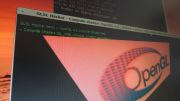Home Utilities Tutorials 3D Demos Graphics Cards Forums About

 GeeXLab
Current version: 0.20.x
>GeeXLab homepage

 FurMark
Current version: 1.20.0
>FurMark homepage

 GPU Caps Viewer
Current version: 1.38.0.0
>GPU Caps Viewer homepage

 GPU Shark
Current version: 0.10.0.5
>GPU Shark homepage

 Blogs
>JeGX's HackLab

 Geeks3D's Articles
>GPU Memory Speed Demystified

>How to Get your Multi-core CPU Busy at 100%

>How To Make a VGA Dummy Plug

>Night Vision Post Processing Filter

 PhysX FluidMark
Current version: 1.5.2
>FluidMark homepage

 TessMark
Current version: 0.3.0
>TessMark homepage

Current version: 0.3.0

 Demoniak3D
Current Version: 1.23.0
>Demoniak3D
>Libraries and Plugins
>Demos
>Codes Samples

3D Graphics Search Engine:By Jérôme 'JeGX' GUINOT - The oZone3D Team
jegx [at] ozone3d [dot] net

Initial draft: September 21, 2005

Translated from french by Jacqueline ESHAGHPOUR - jean25 [at] freesurf [dot] ch
 1 - Introduction2 - Operating3 - Stencil Shadow Volumes in Demoniak3D4 - Rules to be respected5 - Downloads

1 - IntroductionShadows and shading are a particularly active domain in 3D graphics. Shading is the essence for reality in a 3D scene. Shadows are the result of interaction between the light and the objects. Shadows makes it possible to show the effects of depth in the scene and more especially to confer some weight to 3D objects (the observer does not get anymore a "floating impression"). Shadows are classed in two main categories:
In everyday reality, hard shadows do not exist; only soft shadows really exist. But in some cases, and seen from a distance, soft shadows look like hard shadows. All this is fine, but necessitates further explanation. A shadow is considered soft when the passage from the shadowed zone to a lit zone is progressively made. This transition zone is known as penumbra.In figure 1, the soft shadow is clearly visible. On the contrary, a shadow is called "hard" if the passage from the shaded zone to the lit zone is made without transition as shown in figure 2.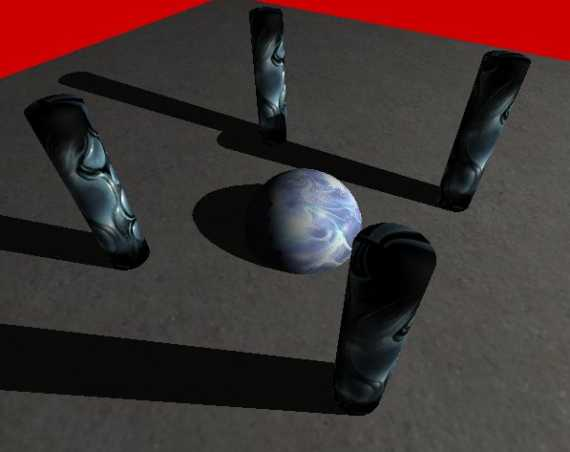Soft shadows are very realistic but are often relatively complex to reproduce in real-time 3D. The algorithms of soft shadows have started to arrive but require tough 3D cards (such as GeForce 6800 GT or better still GeForce 7800 GTX). Hard-shadows, however, are far more rapid to generate and have become extremely common in recent video games. But there is a game which really put hard shadows to the forefront: Doom 3.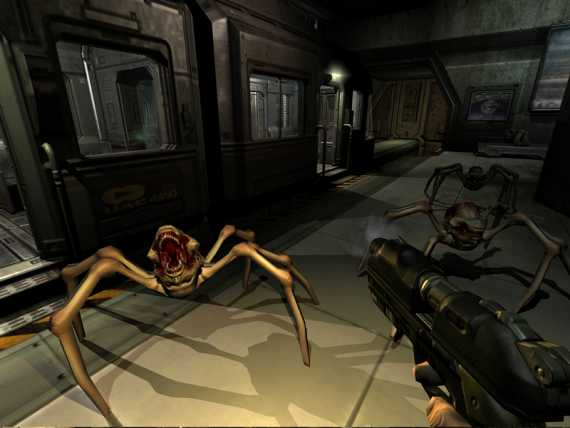fig. 3 - Doom3 - Copyright 2004 Id Software

The technique used in Doom 3 to create these hard shadows bears a precise name: Stencil Shadow Volumes.

The stencil, more known under the name of stencil buffer, is a particular memory zone on the graphics card which is used for all kinds of special effects, amongst which shadow volumes. There is no question of detailing its function as one would need to enter into the heart of OpenGL or of Direct3D to really understand. What it is important to know is that, without the presence of this particular memory zone, shadow volumes would simply not exist.

We will now see how all this works as Demoniak3D runs the Stencil Shadow Volumes...

2 - OperatingIn the global functioning of the stencil shadow volumes, there are three performers:
• the light
Light is generally a positional type source (or OMNI).

The shadow-caster is the shadow thrower: it is the object which will block the light and create the shadow. The shadow-receiver is an object which "receives" the shadow, or to be more precise, it is the object which is totally or partially deprived of light.

The shadows are said to be "volumic" simply because the shadow-caster will create a volume of shadow where the objects therein will not receive any light. Figure 4 shows the volume of shadow generated by a torus: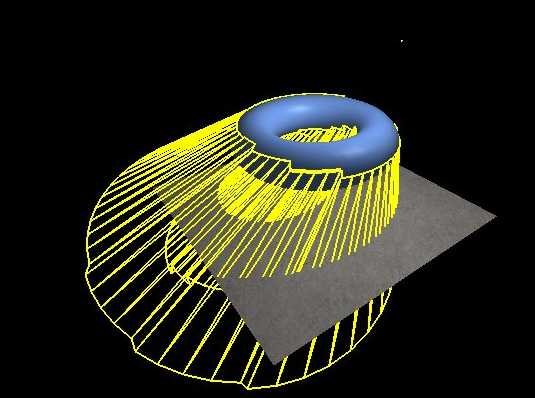The principle of shadow volume is to detect the silhouette of the object from the source light point of view and to project this silhouette in the direction definded by the vector between the light and the object. The projection of the silhouette will form the shadow volume.

3 - Stencil Shadow Volumes in Demoniak3DNow that we have seen the basic principles of shadow volumes, let us get down to the practical side. In Demoniak3Ds operating, shadow volumes only work with meshes or with models as these are the only 3D objects which have a "reality" (virtual nonetheless) in a 3D scene.

Therefore, the use of shadow volumes is easy, as all one has to do is to set to "TRUE" the shadow_caster attribute of the mesh or model nodes. Really simple!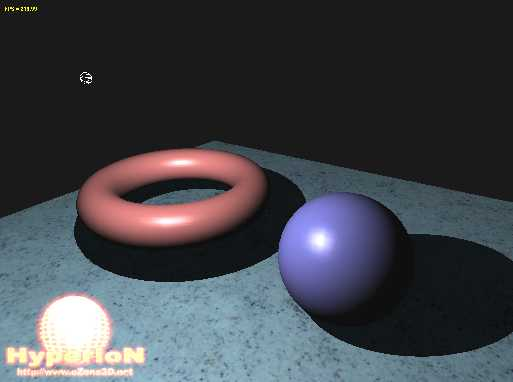fig. 5 - The example project

The following script (script 1) allows one to obtain the scene in figure 5. This scene contains a floor which acts as the shadow-receiver, and two 3D objects (2 models) which are the shadow-casters. The little white wire sphere represents the position of the light source.
```<?xml version="1.0" encoding="ISO-8859-1" standalone="yes"?>
<hyperion version="1.0">
<!--
====== SCENE =======================================
-->
display_fps="TRUE"
vsync="TRUE"
show_ref_grid="FALSE" >

<window_size width="1024" height="768" />
<global_ambient_light r="0.2" g="0.2" b="0.2" />
<background_color r="0.1" g="0.1" b="0.1" />
</scene>

<!--
====== LIGHT ==========================================
-->
<light name="Light_01" render="TRUE" >
<position x="0.0" y="150.0" z="100.0" />
<ambient r="0.2" g="0.2" b="0.2" a="1.0" />
<diffuse r="1.0" g="1.0" b="1.0" a="1.0" />
<specular r="1.0" g="1.0" b="1.0" a="1.0" />
</light>

<!--
====== CAMERA =========================================
-->
<camera name="Main_Camera"  >
<position x="30.0" y="100.0" z="200.0" />
</camera>

<!--
====== FLOOR =========================================
-->
<texture name="floor_tex"
filename="data/Speckles_LightBlue_df.dds" />

<material name="floor_mat" >
<ambient r="0.7" g="0.7" b="0.7" a="1.0" />
<diffuse r="0.9" g="0.9" b="0.9" a="1.0" />
<specular r="0.1" g="0.1" b="0.1" a="1.0" exp="10.0" />
</material>

<mesh name="floor" shape_type="PLANE"
lighting="TRUE" texturing="TRUE"
polygon_mode="SOLID" use_vbo="TRUE" >
<plane x_size="500.0" z_size="500.0"
num_segs_x="20" num_segs_z="20" />
<attach_material name="floor_mat" />

<texture material_name="floor_mat"
texture_name="floor_tex"
texture_unit="0"
u_tile="1.0" v_tile="1.0" />
</mesh>

<!--
====== TORUS MESH =======================================
-->
<material name="mat_torus" specular_exp="60.0">
<ambient r="0.3" g="0.3" b="0.3" a="1.0" />
<diffuse r="0.9" g="0.5" b="0.5" a="1.0" />
<specular r="0.6" g="0.6" b="0.6" a="1.0" />
</material>

<mesh render="TRUE" name="torus" shape_type="TORUS"
lighting="TRUE" use_vbo="TRUE"
improve_specular_highlights="TRUE"

<torus vertex_density="50"/>
<position x="-70.0" y="50.0" z="0.0" />
<attach_material name="mat_torus" />
</mesh>

<!--
====== SPHERE MESH =======================================
-->
<material name="mat_sphere" specular_exp="60.0">
<ambient r="0.3" g="0.3" b="0.3" a="1.0" />
<diffuse r="0.5" g="0.5" b="0.9" a="1.0" />
<specular r="0.6" g="0.6" b="0.6" a="1.0" />
</material>

<mesh render="TRUE" name="sphere" shape_type="SPHERE"
lighting="TRUE" use_vbo="TRUE"
remove_seam="TRUE"  >

<position x="70.0" y="40.0" z="0.0" />
<attach_material name="mat_sphere" />
</mesh>

</hyperion>```

Script 1 does not present any particular difficulty, but it introduces a few novelties and subtleties.

The global ambient light attribute of the scene node allows colour specification of the scenes global ambient light in the absence of all light sources (the light nodes). This specification is important because the shadow volume and the objects (the shadow-receivers) are only lit by this global ambient light. By default, in Demoniak3D, it is initialised at R=0.1, G=0.1 and B=0.1, thus a very low value.

One must also pay particular attention to the choice of the ambient light and to the materials. This is primordial in order to obtain a good self shadow. For memory, the self shadow of an object is the part of the object which does not receive direct (or diffused) light from a light source, and which is solely illuminated by ambient light from this same light source. In the case of the blue sphere in figure 5, the self shadow is the dark part (but which one can distinguish), whereas the blue part (with the whitish reflection) is that which is directly exposed to the light source and which receives diffused (and specular) light.

But the choice of the ambient light and material components is important for yet another reason: to mask and hide the rendering of the silhouettes shadow. Figure 6 shows the rendering of the scene with a bad choice of ambient components, and also a sphere containing few polygons.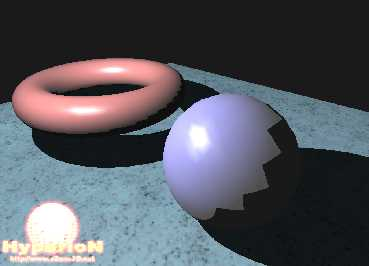In the scene in figure 6, the parameters of the sphere within the mesh node are the following:
```<mesh name="sphere" shape_type="SPHERE" >
</mesh>```

This low polygon density provokes a hideous aliasing effect on the shadow side of the sphere. It is one of the inconveniences of the stencil shadow volumes: the algorithms require a fairly dense polygon object in order to obtain a good silhouette.

The ambient material components of the sphere and of the light, as far as they are concerned, are resolved in the following manner:
```<material name="mat_sphere" >
<ambient r="0.5" g="0.5" b="0.5" a="1.0" />
</material>

<light name="Light_01" >
<ambient r="0.5" g="0.5" b="0.5" a="1.0" />
</light>```

These adjustments accentuate the rendering of the shadow volume on the sphere itself. The first way to ameliorate the visual aspect of the scene in figure 6 is to correctly adjust the ambient components of the light and the spheres material. The following adjustment, while maintaining the low density of the spheres meshing, gives the result seen in figure 7: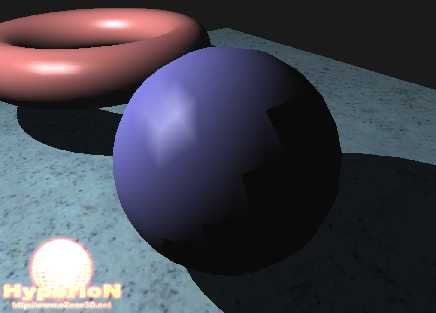Fig.7  Good adjustment of the ambient components

This is becoming excellent! But, if you look closely, you will notice that there still remains an aliasing effect due to the low density of the spheres meshing. (figure 8).Fig.8  One still notices the aliasing effect...

The solution to making this aliasing effect completely disappear is to increase the density of the mesh:
```<mesh name="sphere" shape_type="SPHERE" >
</mesh>```

With these adjustments, the aliasing effect has become imperceptible, as shown in figure 9.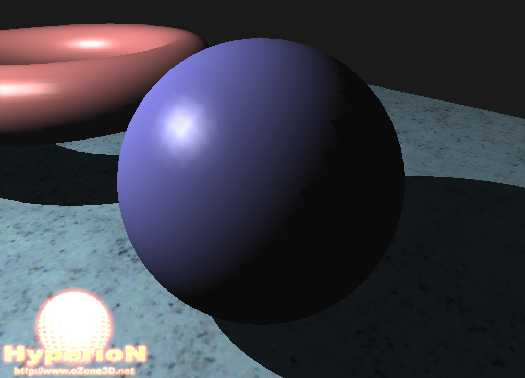Fig.9  The aliasing effect has disappeared

This example shows that in order to obtain a rendering based on stencil shadow volume, the 3D objects must have a certain mesh density, and that the material and light ambient components are adjusted to relatively low values.

This low value of the ambient components is responsible for making scenes which use ambient components rather dark. The game Doom 3 is a perfect example of this!

4 - Obligations to be respectedAs usual in realtime 3D, the most spectacular graphic effects have their price! The most constraining rule concerns the modelling of 3D objects. All 3D models are not candidates to becoming a shadow-caster. In order to project a shadow volume, the meshes forming a model must be closed.

But what is a closed mesh?

Before giving a definition, let us just give a little reminder concerning the structure of the mesh surface. A mesh is a surface composed of triangular polygons. Each triangle represents a surface and is composed of 3 vertices and 3 edges. The simplest mesh one can make is a... triangle.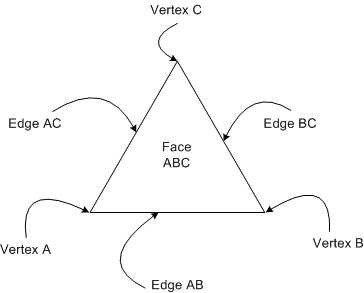Fig.10  The triangle: base component of a mesh

A mesh is said to be closed if each edge is shared by two sides (each edge having two adjacent sides).

The sphere is the perfect example of a closed surface. You cannot find an edge which does not belong to the same side. A plane, to the contrary, is the typical example of an unclosed surface (or open surface!): each edge belongs to the one and only side.

An edge which only has one side is called a crack. Therefore a mesh containing cracks cannot correctly project shadow volumes. This limitation comes from the silhouette detection algorithm which, in order to work, requires closed surfaces.

The model of the teapot, which one can rapidly generate with 3D Studio MAX for example, has cracks. The teapot lid is an unclosed surface. The result of these cracks is seen in figure 11.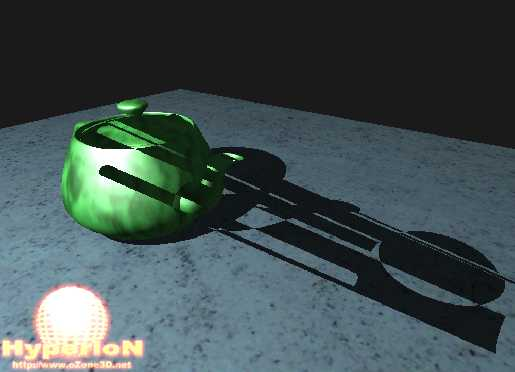Fig.11  Unclosed surfaces cause visual defects

Therefore modelling with the aim of projecting shadow volumes must be made keeping in mind the obligation of a closed surface. For instance, if you wish to have a flat surface (such as a wall) as a shadow-caster, you only need to model a box which is very thin. Seen from a distance, it resembles a plane but for the shadow volume algorithm which sees the model closely, the box is a closed surface.

Another obligation to take into consideration is the number of light sources. The stencil shadow algorithm is by nature a multi-pass algorithm. That means that the whole scene is rendered for each light source. If the scene is filled with polygons, the number of FPS (Frames Per Second) runs the risk of falling drastically! For example: if you have a scene composed of 100000 polygons and 3 light sources, there will be roughly 300000 polygons rendered at each frame.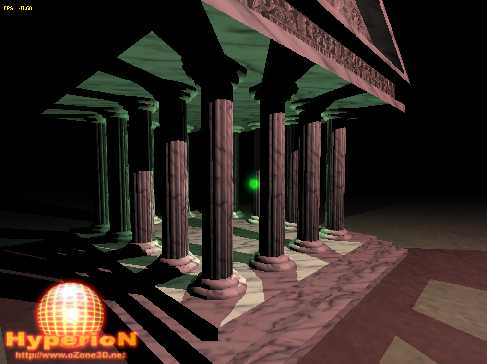Fig.12  Model lit by 2 light sources  Code Sample 70Accompanying project

 GeeXLab demosGLSL - Mesh exploderPhysX 3 cloth demo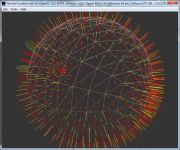Normal visualizer with GS